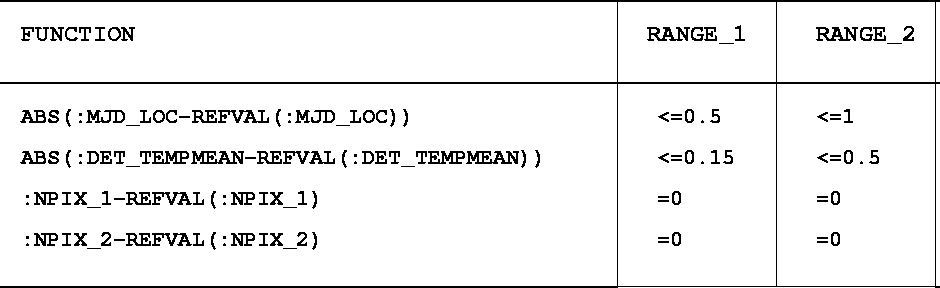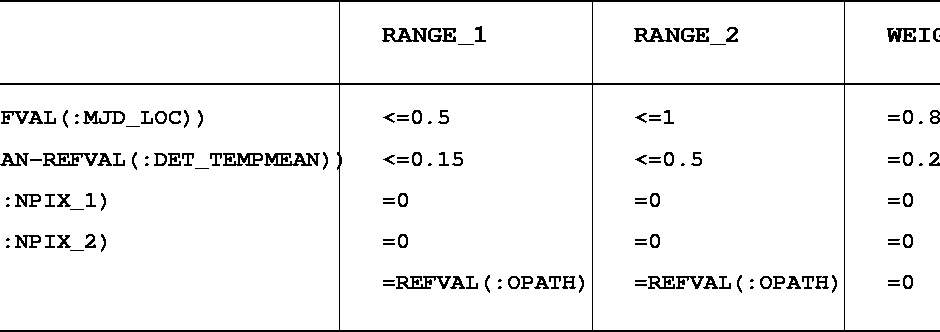Next: Association of Calibration Exposures Up: Association of images Previous: Creation of the Association

An example of selection criteria

Table 15.8 shows a table of association rules that has been created to associate bias exposures with scientific frames. A column labeled :MJD_LOC has been created in the OST and contains a number derived from the Modified Julian Date of the exposure.

• ABS(:MJD_LOC-REFVAL(:MJD_LOC)) is the absolute difference between the time of the scientific frame that is being studied and the time of a dark exposure
• ABS(:DET_TEMPMEAN-REFVAL(:DET_TEMPMEAN)) is the absolute difference between the mean temperature of the detector when the dark exposure was taken and the mean temperature of the detector when the scientific frame was observed.
• :NPIX_1-REFVAL(:NPIX_1) is the difference between the CCD window sizes in the X-direction of a dark exposure and the scientific frame while :NPIX_2-REFVAL(:NPIX_2) is the difference between the CCD window sizes in the Y-direction of a dark exposure and the scientific frame.
Each row of the column labeled :RANGE_1 defines the acceptable first choice" ranges for each of the four functions mentioned previously, and the combination of these four criteria defines the first choice" set of criteria. This may be expressed as:
Given a scientific exposure, select all dark exposures that satisfy:

• The elapsed time between the two exposures is less than 12 hours
• The detector mean temperature did not vary by more than 0.15 degrees between the two exposures
• The two frames have exactly the same size.
The column RANGE_2 defines in the same way the set of second choice" criteria that can be expressed as:
Given a scientific exposure, select all dark exposures that satisfy:
• The elapsed time between the two exposures is less than 12 hours
• The detector mean temperature did not vary by more than 0.5 degrees between the two exposures
• The two frames have exactly the same size.

The graphs of Figure 15.2 illustrate the association of two dark exposures with each of two scientific images from our observing run: susi0006.bdf and susi0009.bdf. The association process when applying the set of criteria mentioned above will find two suitable candidates for susi0006.bdf satisfying the first choice" set of criteria. No first choice" candidates for susi0009.bdf will be found while five second choice" candidates may be selected. Because more importance is assigned to the time difference (weight=0.8) than to the detector temperature difference (weight=0.2), the files susi0001.bdf and susi0002.bdf will be flagged as the best" ones. (Note that the differences are computed in the units involved in the columns involved. In the given example they are degrees kelvin and days, respectively.)
A table of rules (Table 15.9) for associating flat-field exposures has been created following the same scheme. One criterion has to be added to the rules mentioned above: One has to make sure that one associates only frames obtained in the same instrument configuration (i.e in this case with the same filter). Figure 15.3 shows that only one flat field can be associated with the frame susi0006.bdf (for clarity, only the FFs obtained with the same filter as the scientific image are numbered) while three suitable flat-fields may be selected for susi0009.bdfNext: Association of Calibration Exposures Up: Association of images Previous: Creation of the Association
Petra Nass
1999-06-15